# Shapes and Angles

## Objective

Identify and draw parallel lines.

## Common Core Standards

### Core Standards

?

• 4.G.A.1 — Draw points, lines, line segments, rays, angles (right, acute, obtuse), and perpendicular and parallel lines. Identify these in two-dimensional figures.

?

• 3.G.A.1

## Criteria for Success

?

1. Understand that parallel lines are lines that will never intersect, even if they are extended infinitely.
2. Determine whether two lines are parallel or intersecting (even if they are not visibly intersecting) using a straightedge and right-angle template (MP.5).
3. Draw two parallel lines using a straightedge and right-angle template (MP.5, MP.6).
4. Use correct notation to denote parallel lines, e.g., $\overline{AB}\parallel\overline{CD}$, and draw arrows on corresponding parallel sides in a figure.

## Tips for Teachers

?

• Right-angle templates were constructed in Lesson 2 Anchor Task #1 from blank paper.

### Lesson Materials

• Right-angle template (1 per student) — These were constructed in Lesson 2 using blank pieces of paper

#### Fishtank Plus

• Problem Set
• Student Handout Editor
• Vocabulary Package

?

### Problem 1

(Pair up students and give the following image to just one partner in each pair.) Tell your partner how to draw the following shape (without using the word “trapezoid”):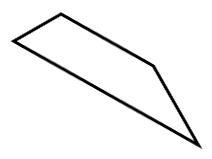### Problem 2

Are these line segments parallel? Be as precise as possible.

 a.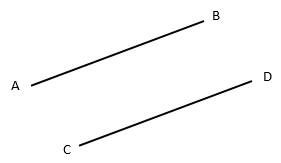b.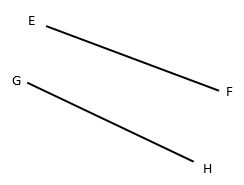#### References

EngageNY Mathematics Grade 4 Mathematics > Module 4 > Topic A > Lesson 4Concept Development Problem 2

Grade 4 Mathematics > Module 4 > Topic A > Lesson 4 of the New York State Common Core Mathematics Curriculum from EngageNY and Great Minds. © 2015 Great Minds. Licensed by EngageNY of the New York State Education Department under the CC BY-NC-SA 3.0 US license. Accessed Dec. 2, 2016, 5:15 p.m..

Modified by Fishtank Learning, Inc.

### Problem 3

Draw parallel line segments as precisely as possible.

#### References

EngageNY Mathematics Grade 4 Mathematics > Module 4 > Topic A > Lesson 4Concept Development Problem 3

Grade 4 Mathematics > Module 4 > Topic A > Lesson 4 of the New York State Common Core Mathematics Curriculum from EngageNY and Great Minds. © 2015 Great Minds. Licensed by EngageNY of the New York State Education Department under the CC BY-NC-SA 3.0 US license. Accessed Dec. 2, 2016, 5:15 p.m..

Modified by Fishtank Learning, Inc.

## Problem Set & Homework

### Discussion of Problem Set

• In #1, how could your right-angle template serve as a guide for identifying parallel lines?
• How do you know if two lines are parallel (#2)?
• In #3, do you agree or disagree with Ms. Glynn? Why? Are there other line segments that are parallel in the figure? How do you know?
• Which shapes in #5 had parallel lines?  Are opposite sides always parallel?
• How do parallel lines differ from perpendicular lines?
• Two segments that don’t intersect must be parallel.  True or false?  Explain.

?

Determine whether the following pairs of lines are parallel, perpendicular, or intersecting.

a.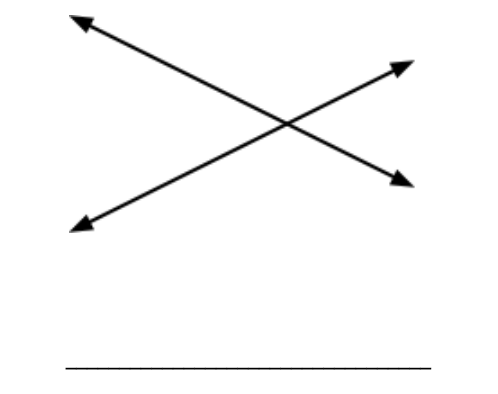b.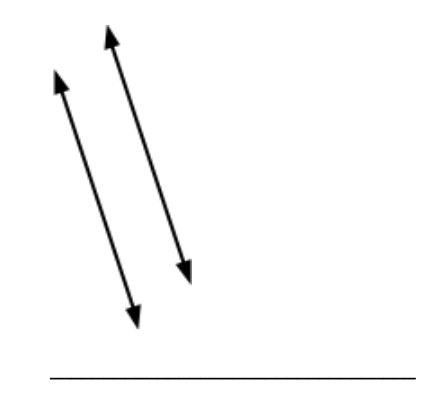c.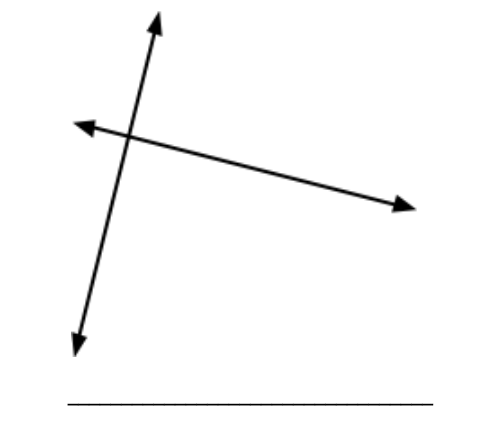d.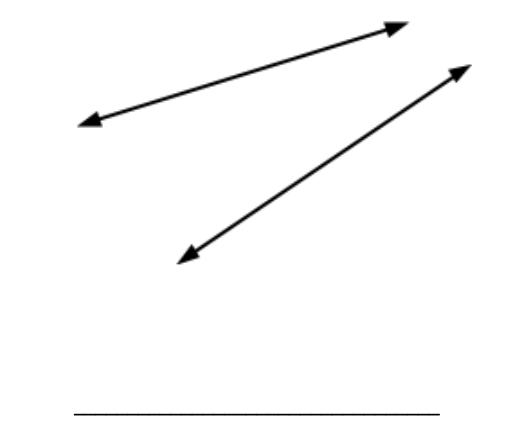#### Mastery Response